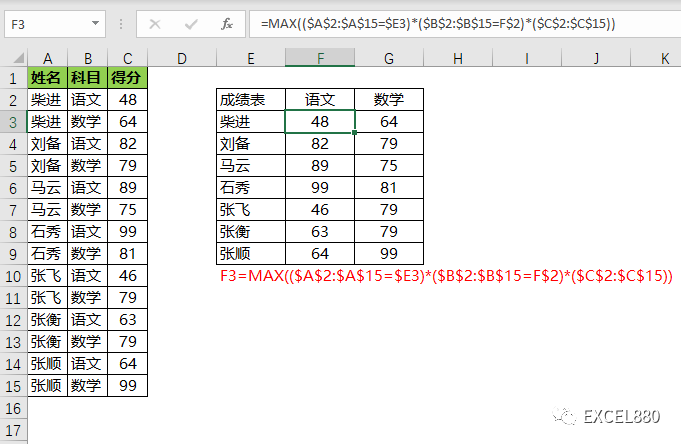# Excel函数进阶：一维表转二维表的5种方法

191 次浏览

XLOOKUP多条件查询

=XLOOKUP($E3&F$2,$A:$A&$B:$B,$C:$C)

$E3&F$2：姓名连接科目作为第一参数，如“柴进语文”。

$A:$A&$B:$B：对应的姓名区域连接科目区域作为第二参数，得到如下数组作为查询区域{“柴进语文”,“柴进数学”,“刘备语文”,“刘备数学”……}

$C:$C：返回C列对应的得分数据。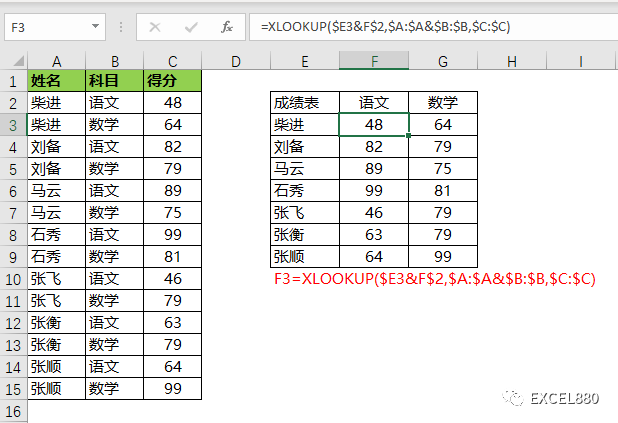FILTER筛选

=FILTER($C$2:$C$15,($A$2:$A$15=$E3)*($B$2:$B$15=F$2))

$C$2:$C$15：被筛选的数据区域。

$A$2:$A$15=$E3：需满足的第一个条件：姓名=柴进。$B$2:$B$15=F$2：需满足的第二个条件：科目=语文。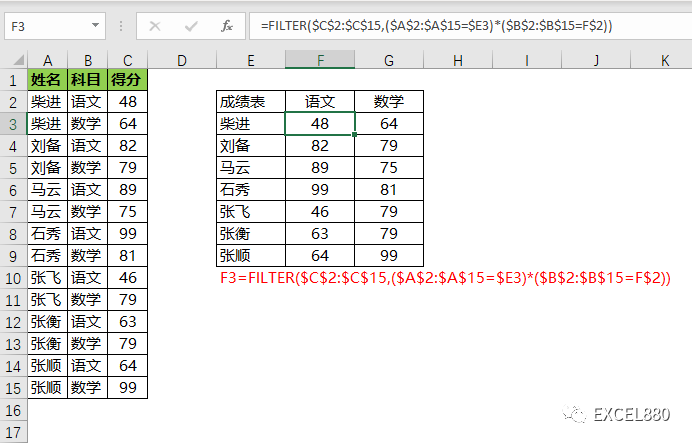SUMIF条件求和

=SUMIFS($C:$C,$A:$A,$E3,$B:$B,F$2)

FILTER是筛选出满足两个条件的得分，SUMIFS是将满足条件的得分求和，有异曲同工之妙。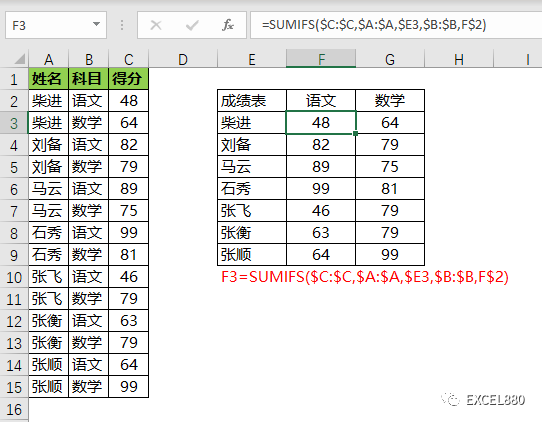VLOOKUP多条件查询

=VLOOKUP($E3&F$2,CHOOSE({1,2},$A:$A&$B:$B,$C:$C),2,0)

VLOOKUP与XLOOKUP属于同类函数，此处VLOOKUP的第一参数仍然是将两个查询条件连接，而CHOOSE的目的是构建一个对应的查询区域作为第二参数，用IF函数也可以实现。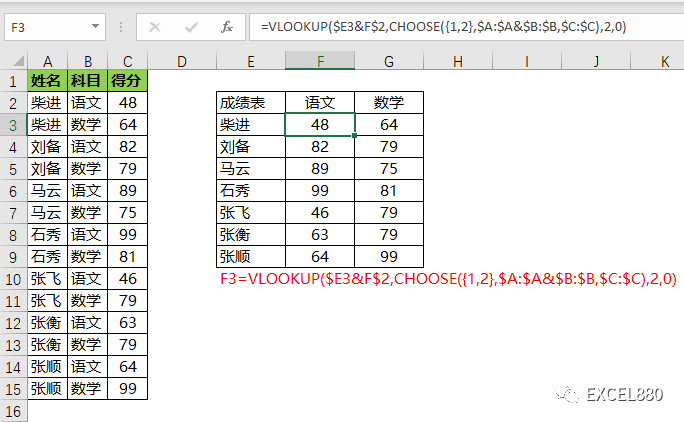MAX结合数组

=MAX(($A$2:$A$15=$E3)*($B$2:$B$15=F$2)*($C$2:$C$15))